# Probabilistic primality test

(diff) ← Older revision | Latest revision (diff) | Newer revision → (diff)

A randomized procedure that takes a natural number as input and attempts to determine whether that number is prime or composite (cf. Prime number). The output of the procedure should be correct with high probability, independently of the number being tested.

It is desirable that such a test run in polynomial time; that is, the number of bit operations used to testfor primality should be no more than a power of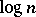. One distinguishes between Las Vegas primality tests, which prove primality, and Monte-Carlo primality tests, which only provide statistical evidence.

Aside from number theory, these tests are of interest in computer science because it is not known whether there is a deterministic polynomial-time algorithm for primality (cf. Complexity theory). In addition, they can be used to select keys for public-key cryptographic systems (cf. Cryptography; Cryptology).

One popular Monte-Carlo test is the strong prime test, studied by several authors. To test an oddfor primality, one first partially factors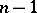as, whereis odd. Then, one chooses a random integerwith, and computes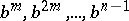modulo. If the sequence is either all's, or contains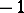, the algorithm says "prime" . In all other cases, the algorithm says "composite" . In the first case, the statement is correct with probability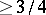, independently of; in the second case, the assertion is always correct. The computation can be done using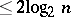multiplications modulo. For the history and more examples of Monte-Carlo tests, see [a3], [a4].

L.M. Adleman and M.-D. Huang have shown, using the Jacobian group of an Abelian variety, that there is a polynomial-time Las Vegas algorithm for primality. They do not consider their algorithm practical. A related heuristic method, based on elliptic curves (cf. Elliptic curve), was designed by A.O.L. Atkin and F. Morain (building on work of S. Goldwasser and J. Kilian), and has been used to find primality proofs for numbers of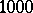decimal digits. For these algorithms, see the overview in [a4] and the original references [a1], [a2].